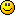#### Topic: New Indicator: Ergodic Indicator

Hi All,

I posted a new indicator called Ergodic Indicator, sometimes recognized as True Strength Index indicator. This indicator is a variation of the Relative Strength Index, developed by William Blau, it uses a differencing function to measure momentum and an averaging function to correlate the momentum to the price trend. In other words, the indicator combines the leading characteristic of a differencing momentum calculation with the lagging characteristic of an averaging function to create an indicator that reflects price direction and is in sync with market turns.Rgds
Denny Imanuel
imanuel.denny@gmail.com

Thank you Denny.

#### Re: New Indicator: Ergodic Indicator

Hi zuijaideai and all forum menber,

Do u have any Ergordic indicator code for meta trader 4, where by the
indicator is same like chart you show on trade station, indicator
with green and red line. Thank.

#### Re: New Indicator: Ergodic Indicator

Dear Guru,

Can some pls convert the below trade station code to MQL4 for meta trader. Thank.

Ergodic Indicator by William Blau

ergodic indicator - text version

* Subject: ergodic indicator - text version
* From: "Barry Silberman" <Barry@xxxxxxxxxxxxxxxxx>
* Date: Tue, 2 Dec 2003 07:07:30 -0800

Here is the code for the ergodic that is very similar to the one used in Woodie's room.

The fellow with the identification of R7 uses a value of 7, 27, 7. That should be equivalent to the values in my indicator of 7, 27, 1, 7.

Here is the text version for the indicator and three functions that can be cut and pasted:

Regards,

Barry Silberman

Type: Function, Name: TXAverage

{TXAverage function

FORMAT: TXAverage(Price,r,s,u) }

Inputs: Price(NumericSeries), r(NumericSimple),s(NumericSimple),
u(NumericSimple);

TXAverage = XAverage(XAverage(XAverage(Price,r),s),u) ;

Type: Function, Name: TSI

{TSI function = True Strength Index by Bill Blau}

{FORMAT: TSI(Price,r,s,u)
Where: r = length of first EMA smoothing of 1 day momentum,
s = length of second EMA smoothing of 1 day smooting,
u = length of third EMA smooting of 1 daymomentum.}

Inputs:
Price(NumericSeries), r(NumericSimple), s(NumericSimple),

u(NumericSimple);

Value1= 100*TXAverage(Price-Price,r,s,u) ; { Numerator }

Value2=TXAverage(AbsValue(Price-Price),r,s,u) ; { Denominator }

If Value2 <> 0 then TSI = Value1 / Value2

Else TSI = 0;

Type: Indicator, Name: Ergodic Indicator

{Ergodic Indicator:}

{from William Blau's book --- "Momentum, Direction and Divergence"
FORMAT: Ergodic_TSI(Price, r, s, u,, SmthLen)
where r = length of first EMA smoothing of 1 day momentum,
s = length of second EMA smoothing of 1 day smooting,
u = length of third EMA smooting of 1 daymomentum,
SmthLen = length of ema signal line.

This is plotting the Average only (value2)}

Inputs: Price(c), r(7), s(27), u(1), Zeroline(0), SmthLen(7),
upcolor_1(green), downcolor_1(red), dotsize(2);

Value1= TSI(Price, r, s, u);

Value2= XAverage(TSI(Price, r, s, u), SmthLen);

If value2 > value2 then
Plot3(Value2, "SigLin", upcolor_1, default, dotsize);

If value2 < value2 then
Plot3(Value2, "SigLin", downcolor_1, default, dotsize);

#### Re: New Indicator: Ergodic Indicator

I get the following error in FSB Pro 1.1:

File name: Ergodic Indicator.cs
ERROR: "Ergodic Indicator" compilation failed.
Line 352 Column 37: An object reference is required for the non-static field, method, or property 'ForexStrategyBuilder.Indicators.Indicator.Bars.get'.
Line 353 Column 37: An object reference is required for the non-static field, method, or property 'ForexStrategyBuilder.Indicators.Indicator.Bars.get'.
Line 354 Column 37: An object reference is required for the non-static field, method, or property 'ForexStrategyBuilder.Indicators.Indicator.Bars.get'.
Line 356 Column 17: An object reference is required for the non-static field, method, or property 'ForexStrategyBuilder.Indicators.Indicator.MovingAverage(int, int, ForexStrategyBuilder.Infrastructure.Enums.MAMethod, double[])'.
Line 357 Column 17: An object reference is required for the non-static field, method, or property 'ForexStrategyBuilder.Indicators.Indicator.MovingAverage(int, int, ForexStrategyBuilder.Infrastructure.Enums.MAMethod, double[])'.
Line 358 Column 17: An object reference is required for the non-static field, method, or property 'ForexStrategyBuilder.Indicators.Indicator.MovingAverage(int, int, ForexStrategyBuilder.Infrastructure.Enums.MAMethod, double[])'.

#### Re: New Indicator: Ergodic Indicator

I got it working now